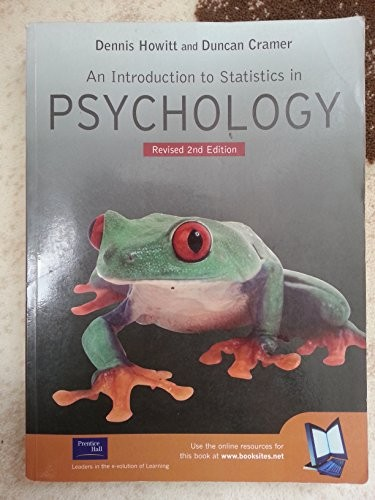# An Introduction to Statistics in Psychology by Dennis Howitt

In Stock
£2.99
Statistics can be tricky, but this revised edition of a guide to statistics for psychology aims to make it much easier. Students at all levels should find the jargon-free style useful. Complex mathematics is kept to a minimum and difficult concepts are explained step-by-step with examples.
Only 2 left

## An Introduction to Statistics in Psychology Summary

### An Introduction to Statistics in Psychology: Revised 2nd Edition by Dennis Howitt

Statistics can be tricky, but this revised edition of a highly effective guide to statistics for psychology makes it much easier. Students at all levels, introductory to advanced, will find the jargon-free style and comprehensive yet accessible approach indispensable. The book is laid out with great visual clarity, making it a highly effective teaching and learning tool.

Complex mathematics is kept to a minimum and concepts that are often difficult to grasp are explained step-by-step using a wide variety of examples.

### Why buy from World of BooksOur excellent value books literally don't cost the earthFree delivery in the UKEvery used book bought is one saved from landfill

Introduction
PART 1: DESCRIPTIVE STATISTICS
1. Why You Need Statistics: Types of Data
2. Describing Variables: Tables and Diagrams
3. Describing Variables Numerically: Averages, Variation and Spread
4. Shapes of Distributions of Scores
5. Standard Deviation: The Standard Unit of Measurement in Statistics
6. Relationships Between Two or More Variables: Diagrams and Tables
7. Correlation Coefficients: Pearson Correlation and Spearman's Rho
8. Regression: Prediction with Precision
PART 2: SIGNIFICANCE TESTING
9. Samples and Populations: Generalising and Inferring
10. Statistical Significance for the Correlation Coefficient: A Practical Introduction to Statistical Inference
11. Standard Error: The Standard Deviation of the Means of Samples
12. T-Test: Comparing Two Samples of Correlated /Related Scores
13. The T-Test Comparing Two Groups of Unrelated/Uncorrelated Scores
14. Chi-Squared: Differences Between Samples of Frequency Data
15. Probability
16. Reporting Significance Levels Succinctly
17. One-Tailed Versus Two-Tailed Significance Testing
18. Ranking Tests: Non-Parametric Statistics
PART 3: INTRODUCTION TO ANALYSIS OF VARIANCE
19. The Variance Ratio Test: The F-Ratio to Compare Two Variances
20. Analysis of Variance (ANOVA): Introduction to the One-Way Unrelated or Uncorrelated Anova
21. Analysis of Variance for Correlated Scores or Repeated Measures
22. Two-Way Analysis of Variance for Unrelated/Uncorrelated Scores: Two Experiments for the Price of One?
23. Multiple Comparisons in ANOVA: Just Where do the Differences Lie?
24. More on the Analysis of Variance (ANOVA): Special Variants of the Approach
Chapter 25. Statistics and the Analysis of Experiments
PART 4: MORE ADVANCED CORRELATIONAL STATISTICS
Chapter 26. Partial Correlation: Spurious Correlation, Third Variables, Suppresser Variables
Chapter 27. Factor Analysis: Simplifying Complex Data
Chapter 28. Multiple Regression and Multiple Correlation
Chapter 29. Path Analysis
Chapter 30. The Analysis of a Questionnaire/Survey Project
Chapter 31. Statistical Power Analysis: Do My Findings Matter?
Chapter 32. Meta-analysis: Combining and Exploring Statistical Findings from Previous Research
Chapter 33. Reliability in Scales and Measurement: consistency and agreement
Chapter 34. Confidence Intervals
Chapter 35. The Analysis of Complex Contingency Tables: Log-Linear Methods
Appendix A. Testing for Excessively Skewed Distributions
Appendix B. Large Sample Formulae for the Non-Parametric Tests
Appendix C. Extended Tables of Significance for the Pearson Correlation Coefficient
Appendix D. Table of Significance for the Spearman Correlation Coefficient
Appendix E. Extended Table of Significance for the t-Test
Appendix F. Table of Significance for Chi-Square
Appendix G. Extended Table of Significance for the Sign Test
Appendix H. Table of Significance for the Wilcoxon Matched Pairs Test
Appendix I. Table of Significance for the Mann-Whitney U-Test
Appendix J. Table of Significant values for the F-Distribution
Appendix K. Table of Significant Values of t- When Making Multiple t-Tests
Appendix
References
Index

GOR001394201
An Introduction to Statistics in Psychology: Revised 2nd Edition by Dennis Howitt
Dennis Howitt
Used - Very Good
Paperback
Pearson Education (US)
2002-08-07
496
0131399829
9780131399822
N/A
Book picture is for illustrative purposes only, actual binding, cover or edition may vary.
This is a used book - there is no escaping the fact it has been read by someone else and it will show signs of wear and previous use. Overall we expect it to be in very good condition, but if you are not entirely satisfied please get in touch with us.